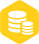# 使用AT89S51单片机制作红外遥控器的资料和源代码详细说明

1.1w人已加入

### 描述

一般红外电视遥控器的输出都是用编码后串行数据对38～40kHz的方波进行脉冲幅度调制而产生的。

当发射器按键按下后，即有遥控码发出，所按的键不同遥控编码也不同。这种遥控码具有以下特征：

采用脉宽调制的串行码，以脉宽为0.565ms、间隔0.56ms、周期为1.125ms的组合表示二进制的“0”；以脉宽为0.565ms、间隔1.685ms、周期为2.25ms的组合表示二进制的“1”。

上述“0”和“1”组成的32位二进制码经38kHz的载频进行二次调制，然后再通过红外发射二极管产生红外线向空间发射。一般电视遥控器的遥控编码是连续的32位二进制码组，其中前16位为用户识别码，能区别不同的红外遥控设备，防止不同机种遥控码互相干扰。后16位为8位的操作码和8位的操作反码，用于核对数据是否接收准确。

根据红外编码的格式，发送数据前需要先发送9ms的起始码和4.5ms的结果码。

接收方一般使用TL0038一体化红外线接收器进行接收解码，当TL0038接收到38kHz红外信号时，输出端输出低电平，否则为高电平。所以红外遥控器发送红外信号时，参考上面遥控串行数据编码波形图，在低电平处发送38kHz红外信号，高电平处则不发送红外信号。

C51程序代码：

#include 《AT89X51.h》

static bit OP; //红外发射管的亮灭

static unsigned int count; //延时计数器

static unsigned int endcount; //终止延时计数

static unsigned char flag; //红外发送标志

void SendIRdata（char p_irdata）;

void delay（）;

void main（void）

{

count = 0;

flag = 0;

OP = 0;

P3_4 = 0;

EA = 1; //允许CPU中断

TMOD = 0x11; //设定时器0和1为16位模式1

ET0 = 1; //定时器0中断允许

TH0 = 0xFF;

TL0 = 0xE6; //设定时值0为38K 也就是每隔26us中断一次

TR0 = 1;//开始计数

do{

delay（）;

SendIRdata（12）;

}while（1）;

}

//定时器0中断处理

void timeint（void） interrupt 1

{

TH0=0xFF;

TL0=0xE6; //设定时值为38K 也就是每隔26us中断一次

count++;

if （flag==1）

{

OP=~OP;

}

else

{

OP = 0;

}

P3_4 = OP;

}

void SendIRdata（char p_irdata）

{

int i;

char irdata=p_irdata;

//发送9ms的起始码

endcount=223;

flag=1;

count=0;

do{}while（count《endcount）;

//发送4.5ms的结果码

endcount=117

flag=0;

count=0;

do{}while（count《endcount）;

//发送十六位地址的前八位

for（i=0;i《8;i++）

{

//先发送0.56ms的38KHZ红外波（即编码中0.56ms的低电平）

endcount=10;

flag=1;

count=0;

do{}while（count《endcount）;

//停止发送红外信号（即编码中的高电平）

if（irdata-（irdata/2）*2） //判断二进制数个位为1还是0

{

endcount=41; //1为宽的高电平

}

else

{

endcount=15; //0为窄的高电平

}

flag=0;

count=0;

do{}while（count《endcount）;

irdata=irdata》》1;

}

//发送十六位地址的后八位

for（i=0;i《8;i++）

{

endcount=10;

flag=1;

count=0;

do{}while（count《endcount）;

if（irdata-（irdata/2）*2）

{

endcount=41;

}

else

{

endcount=15;

}

flag=0;

count=0;

do{}while（count《endcount）;

irdata=irdata》》1;

}

//发送八位数据

irdata=p_irdata;

for（i=0;i《8;i++）

{

endcount=10;

flag=1;

count=0;

do{}while（count《endcount）;

if（irdata-（irdata/2）*2）

{

endcount=41;

}

else

{

endcount=15;

}

flag=0;

count=0;

do{}while（count《endcount）;

irdata=irdata》》1;

}

//发送八位数据的反码

irdata=~p_irdata;

for（i=0;i《8;i++）

{

endcount=10;

flag=1;

count=0;

do{}while（count《endcount）;

if（irdata-（irdata/2）*2）

{

endcount=41;

}

else

{

endcount=15;

}

flag=0;

count=0;

do{}while（count《endcount）;

irdata=irdata》》1;

}

endcount=10;

flag=1;

count=0;

do{}while（count《endcount）;

flag=0;

}

void delay（）

{

int i，j;

for（i=0;i《400;i++）

{

for（j=0;j《100;j++）

{

}

}

}

因为只是实验，所以顾不得外形的美观了，呵呵，不好意思献丑了！

照片中下面的是我电视卡所附的遥控器，中间的为电视卡红外接收头，上面的电路可用来“代替”原配遥控器来控制电视卡电视频道……×
20